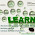## Thursday, 29 May 2014

### Eco-Stats Lab May 2014: Longitudinal data

In this week’s lab we will learn about analysing longitudinal data, that is, data collected over time for each study unit (e.g. subject or site).

Standard (generalised) linear models assume all observations are independent, which is typically not reasonable if repeated measures have been taken over time.  Data collected for one site at times close together will be more similar than data for different sites, or for times further apart. This induces correlation in the data, which we must account for to obtain valid inference.

Normal data

library(lme4)
library(nlme)
library(MASS)

data(BodyWeight)

#scatterplot by Diet
plot(weight~Time,col=Diet,data=BodyWeight)

Rat.lm0=lm(weight~Time,data=BodyWeight)
Rat.lm=lm(weight~Time+Diet,data=BodyWeight)
summary(Rat.lm)
anova(Rat.lm0,Rat.lm)
plot(Rat.lm,which=1)
plot(Rat.lm\$fitted,Rat.lm\$residuals,col=BodyWeight\$Rat)

Problem:
The effects of ignoring dependence vary with the type of dependence but in many cases you can expect
• Standard errors are underestimated, giving “false confidence”
• T-statistics will be overestimated, and regression coefficients that appear significant may not be

Solution 1 – mixed effects model with random intercept and slope

#plot data by Rat
interaction.plot(BodyWeight\$Time, BodyWeight\$Rat, BodyWeight\$weight)

#model with random effects for intercept and slope
Rat.mixed0<- lmer(weight ~ Time + (1 + Time | Rat) , data = BodyWeight)
Rat.mixed<- lmer(weight ~Time +Diet+ (1 + Time | Rat) , data = BodyWeight)
anova(Rat.mixed0,Rat.mixed)

#There seems to be an effect of diet.

summary(Rat.mixed)
plot(Rat.mixed)
plot(fitted(Rat.mixed),residuals(Rat.mixed),col=BodyWeight\$Rat)

This analysis assumes that after fitting a line for each subject, the observations for each subject have the same correlation, regardless of how far away they are in time.  This is not generally realistic; observations closer to each other in time might be more correlated than those further apart.

We can’t use lme4 for analysis which incorporates more flexible correlation structure, we will need to use library(nlme). The autocorrelation structure most commonly used for data correlated in time is autoregressive AR(1). It assumes data points close in time are more strongly correlated than those further apart in time.

Solution 2 – mixed effects model with flexible correlation structures

Rat.lme0 <-lme(weight ~  Time + Diet, random = ~ Time  | Rat, data = BodyWeight)
Rat.lme1 <-lme(weight ~  Time + Diet, random = ~ Time  | Rat, corr=corAR1(, form= ~ Time| Rat), data = BodyWeight)

AIC(Rat.lme0,Rat.lme1 )

#We can see that the AR1 correlation structure seems to work better as the AIC is smaller for this model.

Non Normal Data

What about non normal data? Well we can use the same set up as before in lme4, but now using the glmer function.

data(epil)
interaction.plot(epil\$period, epil\$subject, epil\$y)
interaction.plot(epil\$period, epil\$subject, log(epil\$y+1),col=epil\$subject)

#model with random effects for intercept and slope
Epil.mixed0<- glmer(y ~1 + period + (1 + period | subject) , data = epil,family=poisson)
Epil.mixed<- glmer(y ~1 + period +age+ (1 + period | subject) , data = epil,family=poisson)

# whoops, warning about convergence, let's not worry about it, it's not a problem in this case
# read http://stackoverflow.com/a/21370041 and http://stats.stackexchange.com/a/99719 if you have a similar problem

anova(Epil.mixed0,Epil.mixed)

#There seems to be no effect of age.

What if we want an AR(1) correlation structure instead? Well, it is more complicated, some options are glmmPQL in the MASS package, and geeglm in the geepack package. glmmPQL uses the lme function above, and has very similar syntax. geeglm does not fit quite the same model as lme4, but can also be used if you would like to fit an AR(1)  structure, or other more flexible correlation structures.

1.The interaction plot was really useful for working out what to do with the rat data.
interaction.plot(BodyWeight\$Time, BodyWeight\$Rat, BodyWeight\$weight)
In this plot there are some rats which had generally larger or small er weights (hence the need for an intercept by rat) and also a suggestion that some lines had different slopes (hence the need for a slope effect by rat, Time|rat)

2.to visualise whether you need a structured correlation matrix, for equally spaced observations in time, you could try:
ACF(Rat.lme0)
Our observations weren't quite equally spaced in time, but they nearly are, so this is only a little bit naughty. Interesting nothing much popped up for short-range dependence here: an autocorrelation of 0.1 at lag 1 doesn't seem to be screaming at you that there is heaps of autocorrelation, maybe the fact that it steadily decreases on increasing lag times towards 5 is meaningful??

1.ACF(Rat.lme0,alpha=0.05)
to put confidence bands on the plot. They fan out because when estimating correlation at longer lags there are less observations for estimating the correlation (e.g. lag 10, there is only one pair of values for each rat, the first and last)
This should be interpreted with some caution though because there is one observation time in the data that it is not the same time lag as everything else which messes with things a bit - measurements are weekly except for an extra one at day 44 for some reason, a day after the weekly measurement at day 43.

3.Data Modelling Online Training, ONLINE TRAINING – IT SUPPORT – CORPORATE TRAINING http://www.21cssindia.com/courses/datamodelling-online-training-24.html The 21st Century Software Solutions of India offers one of the Largest conglomerations of Software Training, IT Support, Corporate Training institute in India - +919000444287 - +917386622889 - Visakhapatnam,Hyderabad Data Modelling Online Training, Data Modelling Training, Data Modelling, Data Modelling Online Training| Data Modelling Training| Data Modelling| Courses at 21st Century Software Solutions
Talend Online Training -Hyperion Online Training - IBM Unica Online Training - Siteminder Online Training - SharePoint Online Training - Informatica Online Training - SalesForce Online Training - Many more… | Call Us +917386622889 - +919000444287 - contact@21cssindia.com
Visit: http://www.21cssindia.com/courses.html